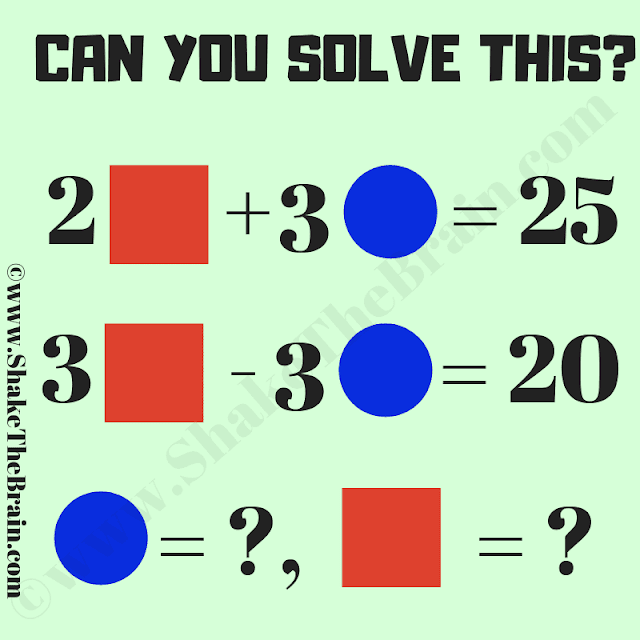Engage students with a captivating maths picture brain teaser: an algebra problem. Challenge their problem-solving skills and unveil the answer.

This Maths Picture Equations Brain Teaser is for school-going, students. Solving this Math Brainteaser will help you to test and improve your maths skills. In this math brain teaser, you are shown some algebraic equations. Solve these simultaneous equations to find the value of the Circle and Square.Can you solve this Maths Picture Brain Teaser?

The answer to this "Maths Picture Equation Brain Teaser", can be viewed by clicking the answer button.